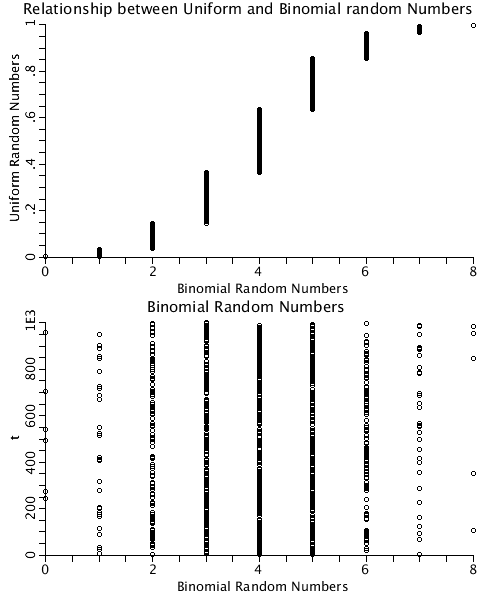# BinomialRandomNumbers

Model number
0389

Generate Binomial random numbers.

## Description

```     The binomial distribution is calculated by first calculating the
natural logarithm of each term and then applying the exponential function
to the result. (See java source code for real function Binomial).
Generate each binomial random number using a uniform random number
as a search key into the cumulative density function to locate the integer
value. (See java source code for real function BinomialRandom.)
As a check for this  calculation, count the number of random numbers
for each value of k using real function BinomialRandomCnt.
The binomial Distribution is plotted (See plot page BinomialDist) with
the counts of the random numbers normalized by the total number of random
numbers generated. For p=0.5, the Bernoulli numbers are calculated as a
check.
It is not necessary to include or call the BinomialRandomCnt java
code if the checking routine is not counted.
The program is demonstrated for n=8, p=0.5. As t.max is increased,
the difference between Binomial(k,n,p) and the distribution of the
generated binomial random numbers equal to k becomes smaller and smaller.
Try setting t.max to 1.0E5```## Equations

None.

The equations for this model may be viewed by running the JSim model applet and clicking on the Source tab at the bottom left of JSim's Run Time graphical user interface. The equations are written in JSim's Mathematical Modeling Language (MML). See the Introduction to MML and the MML Reference Manual. Additional documentation for MML can be found by using the search option at the Physiome home page.